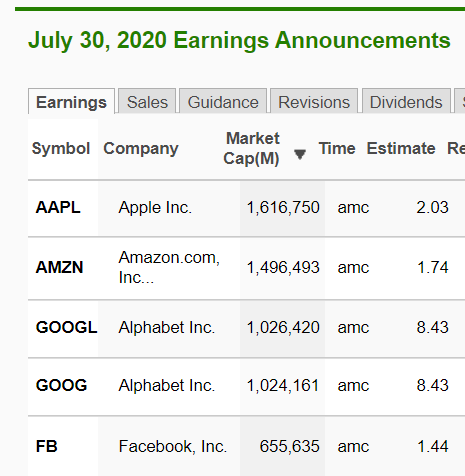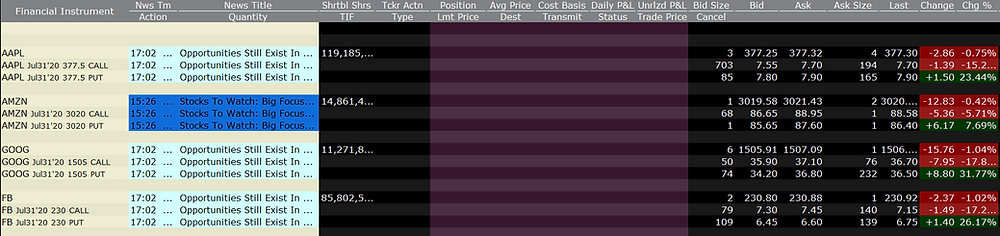Search
• Dani

# Implied move after Earnings

Today after the market close, 4 of the biggest tech companies are reporting earnings:So what can we learn from the options prices about the market expectations for the moves after the earnings are out.

In order to calculate that we need to look at the price of a 1 day at the money straddle.

If someone buys AAPL straddle for 15.55\$ (Call = 7.65 & Put = 7.9) then the break-even point for him is a move of 15.55\$ to either direction. This equals a move of 4.1%, thus if AAPL will move more than that (above 392.85 or under 361.75), the straddle buyer will profit, and if it will move less than that, he will lose.

The options prices show us where is the equilibrium between the buyers and the sellers of options according to their expectation about the earnings.So we can calculate the following:

AAPL: BE move of 4.1%.

AMZN : BE move of 5.8% (175/3020).

GOOG: BE move of 4.9% (73.2/1506) .

FB: BE move of 6% (14/230.85).

The stocks will have time until tomorrow's close to make that move.

If you have a directional bias, you can buy just one side of the straddle and BE at half the above expected move.